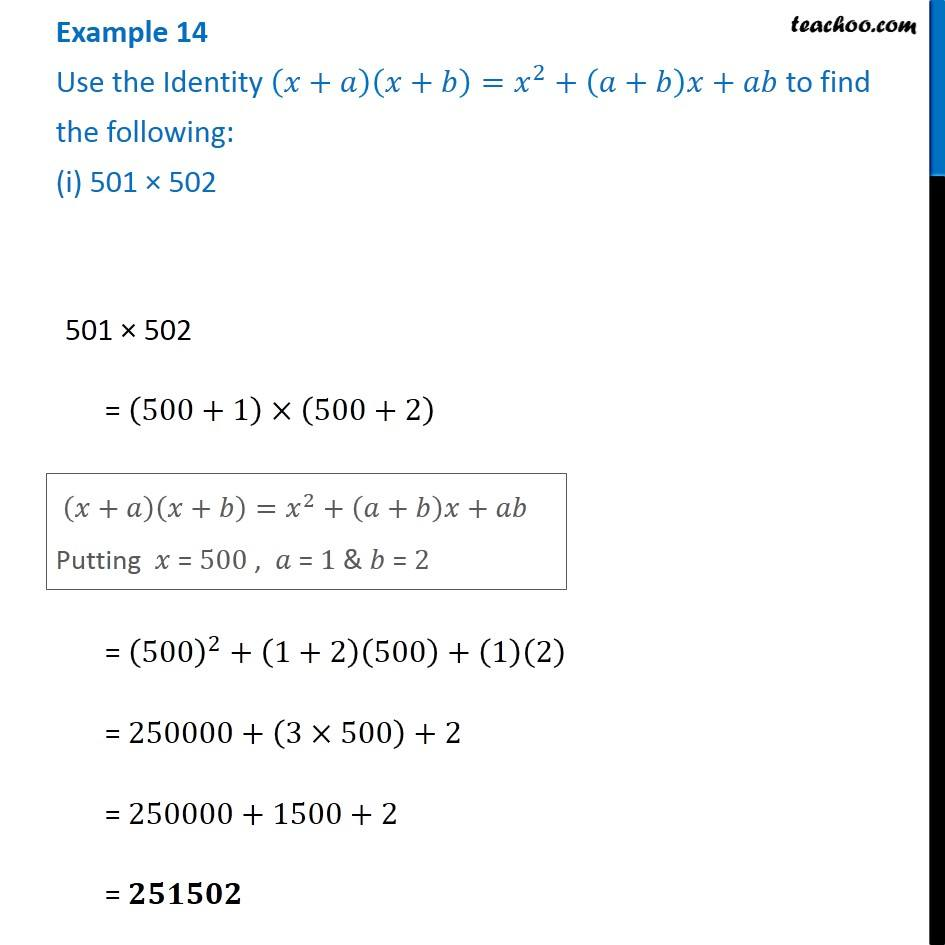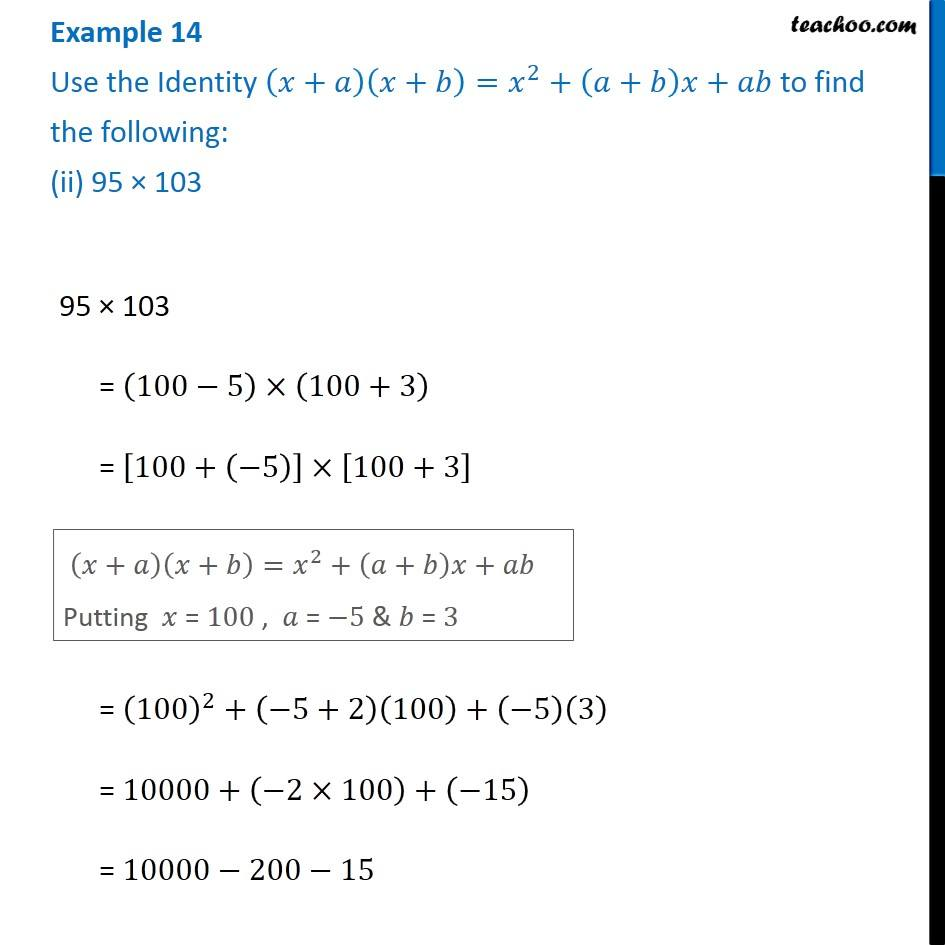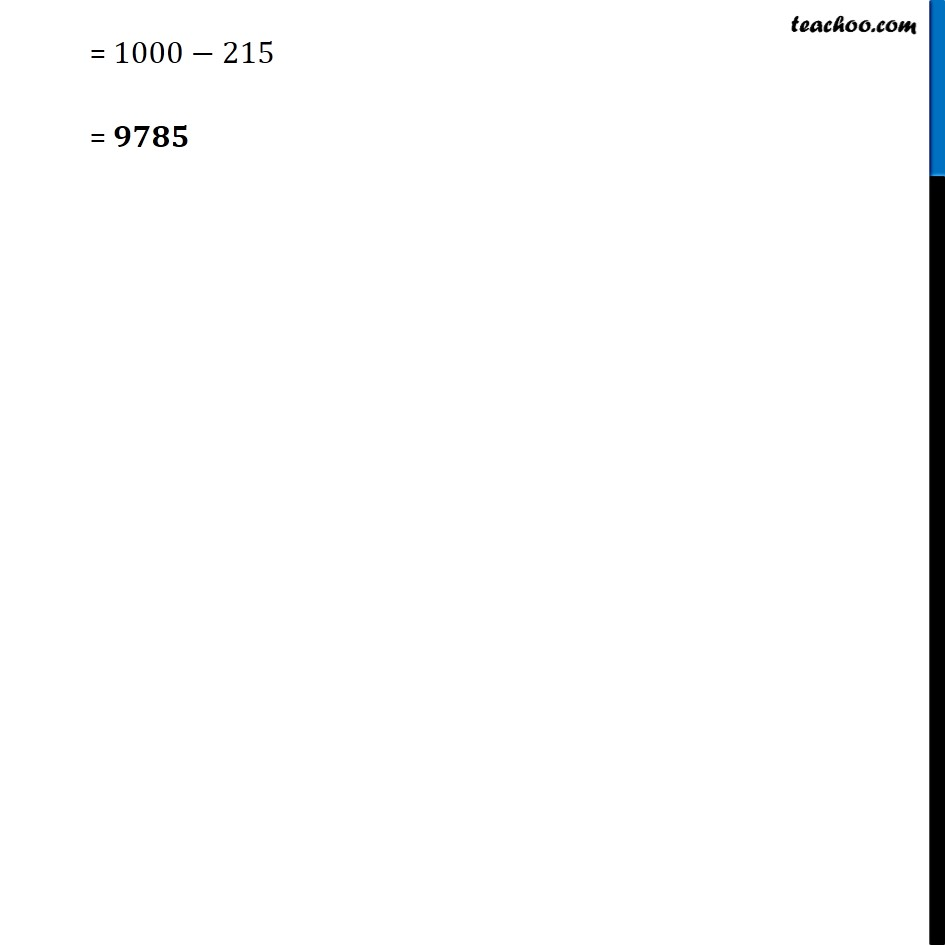1. Chapter 9 Class 8 Algebraic Expressions and Identities
2. Serial order wise
3. Examples

Transcript

Example 14 Use the Identity (𝑥+𝑎)(𝑥+𝑏)=𝑥^2+(𝑎+𝑏)𝑥+𝑎𝑏 to find the following: (i) 501 × 502 501 × 502 = (500+1)×(500+2) (𝑥+𝑎)(𝑥+𝑏)=𝑥^2+(𝑎+𝑏)𝑥+𝑎𝑏 Putting 𝑥 = 500 , 𝑎 = 1 & 𝑏 = 2 = (500)^2+(1+2)(500)+(1)(2) = 250000+(3×500)+2 = 250000+1500+2 = 𝟐𝟓𝟏𝟓𝟎𝟐 Example 14 Use the Identity (𝑥+𝑎)(𝑥+𝑏)=𝑥^2+(𝑎+𝑏)𝑥+𝑎𝑏 to find the following: (ii) 95 × 103 95 × 103 = (100−5)×(100+3) = [100+(−5)]×[100+3] (𝑥+𝑎)(𝑥+𝑏)=𝑥^2+(𝑎+𝑏)𝑥+𝑎𝑏 Putting 𝑥 = 100 , 𝑎 = −5 & 𝑏 = 3 = (100)^2+(−5+2)(100)+(−5)(3) = 10000+(−2×100)+(−15) = 10000−200−15 = 1000−215 = 𝟗𝟕𝟖𝟓

Examples